# 2.3   Recursion

The idea of calling one function from another immediately suggests the possibility of a function calling itself. The function-call mechanism in Java supports this possibility, which is known as recursion.

The "Hello, World" for recursion is the factorial function, which is defined for positive integers n by the equation
$$n! = n \times (n-1) \times (n-2) \times \; \ldots \; \times 2 \times 1$$
The quantity n! is easy to compute with a for loop, but an even easier method in Factorial.java is to use the following recursive function:
 public static long factorial(int n) { if (n == 1) return 1; return n * factorial(n-1); } 
We can trace this computation in precisely the same way that we trace any sequence of function calls.
 factorial(5) factorial(4) factorial(3) factorial(2) factorial(1) return 1 return 2*1 = 2 return 3*2 = 6 return 4*6 = 24 return 5*24 = 120 

Our factorial() implementation exhibits the two main components that are required for every recursive function.

• The base case returns a value without making any subsequent recursive calls. It does this for one or more special input values for which the function can be evaluated without recursion. For factorial(), the base case is n = 1.

• The reduction step is the central part of a recursive function. It relates the value of the function at one (or more) input values to the value of the function at one (or more) other input values. Furthermore, the sequence of input values values must converge to the base case. For factorial(), the value of n decreases by 1 for each call, so the sequence of input values converges to the base case.

## Mathematical induction.

Recursive programming is directly related to mathematical induction, a technique for proving facts about natural numbers. Proving that a statement involving an integer n is true for infinitely many values of n by mathematical induction involves the following two steps:

• The base case: prove the statement true for some specific value or values of n (usually 0 or 1).

• The induction step: assume that the statement to be true for all positive integers less than n, then use that fact to prove it true for n.
Such a proof suffices to show that the statement is true for infinitely many values of n: we can start at the base case, and use our proof to establish that the statement is true for each larger value of n, one by one.

## Euclid's algorithm.

The greatest common divisor (gcd) of two positive integers is the largest integer that divides evenly into both of them. For example, the gcd(102, 68) = 34.

We can efficiently compute the gcd using the following property, which holds for positive integers p and q:

If p > q, the gcd of p and q is the same as the gcd of q and p % q.

The static method gcd() in Euclid.java is a compact recursive function whose reduction step is based on this property.

 gcd(1440, 408) gcd(408, 216) gcd(216, 192) gcd(192, 24) gcd(24, 0) return 24 return 24 return 24 return 24 return 24 

## Towers of Hanoi.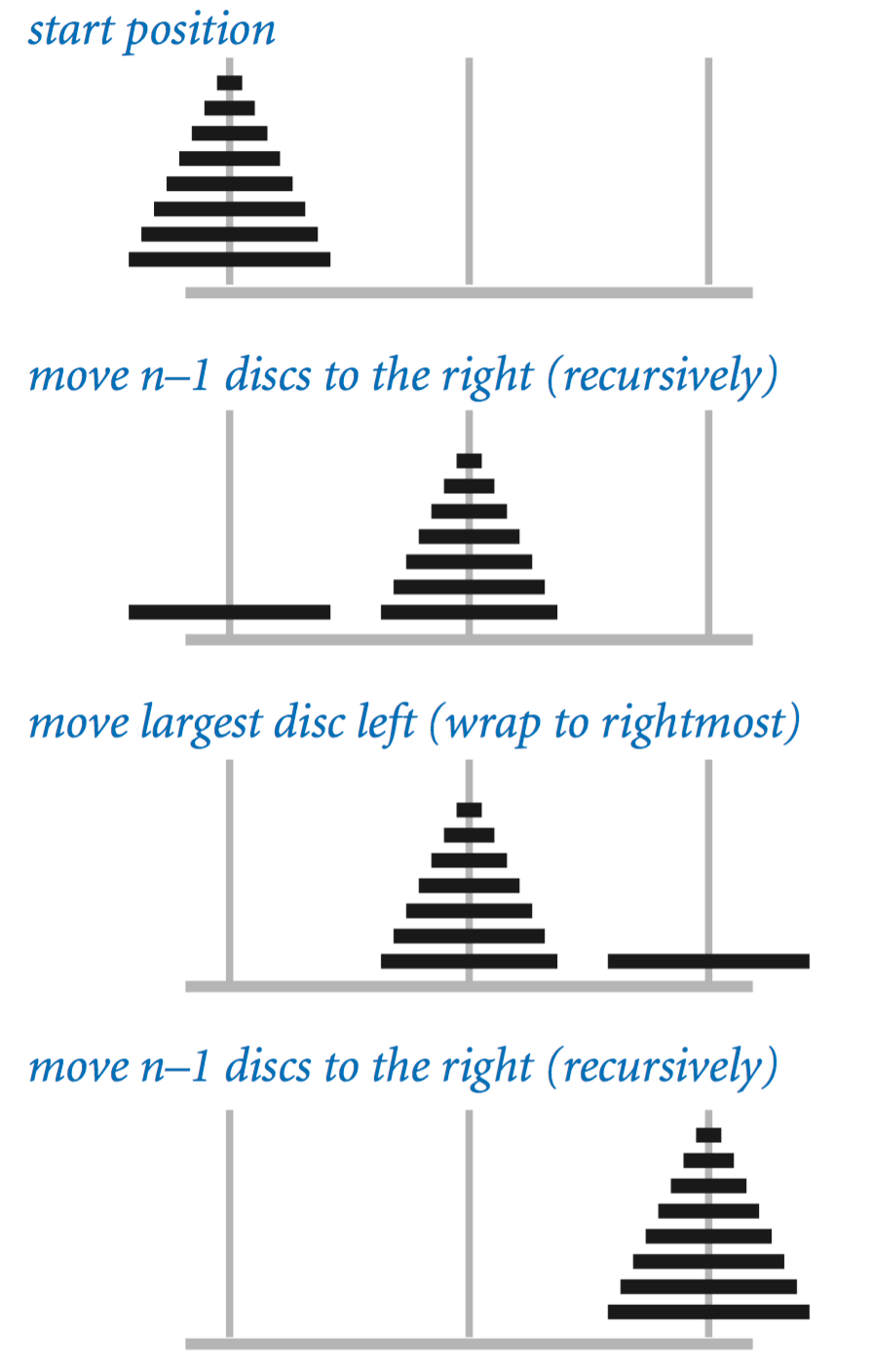In the towers of Hanoi problem, we have three poles and n discs that fit onto the poles. The discs differ in size and are initially stacked on one of the poles, in order from largest (disc n) at the bottom to smallest (disc 1) at the top. The task is to move all n discs to another pole, while obeying the following rules:
• Move only one disc at a time.
• Never place a larger disc on a smaller one.

Recursion provides just the plan that we need: First we move the top n−1 discs to an empty pole, then we move the largest disc to the other empty pole, then complete the job by moving the n−1 discs onto the largest disc. TowersOfHanoi.java is a direct implementation of this strategy.

## Exponential time.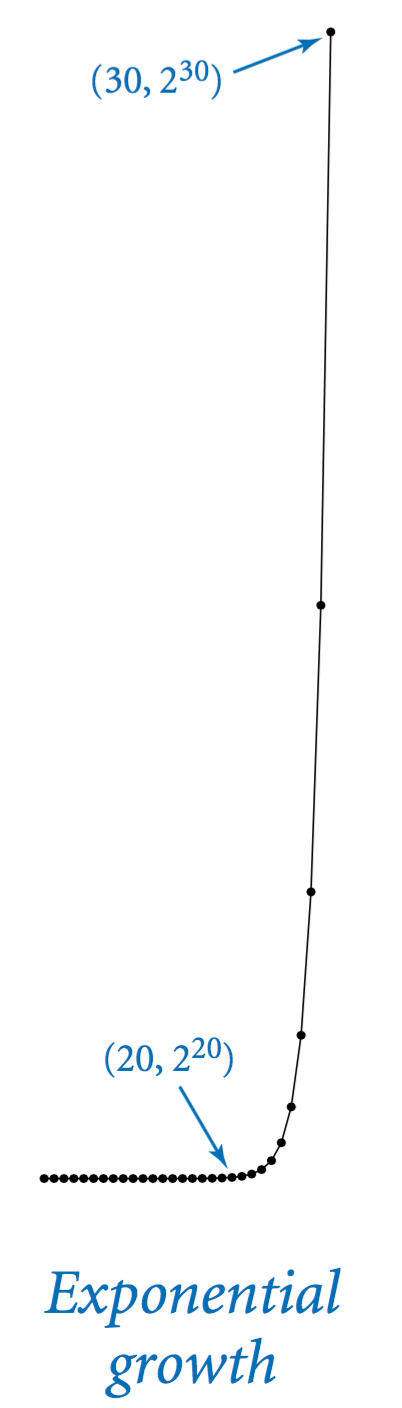Let T(n) be the number of move directives issued by TowersOfHanoi.java to move n discs from one peg to another. Then, T(n) must satisfy the following equation:
$$T(n) = 2T(n-1) + 1 \text{ for } n > 1, \text{ with } T(1) = 1$$

Such an equation is known in discrete mathematics as a recurrence relation. We can often use them to derive a closed-form expression for the quantity of interest. For example, T(1) = 1, T(2) = 3, T(3) = 7, and T(4) = 15. In general, T(n) = 2n − 1. Assuming the monks move discs at the rate of one per second, it would take them more 5.8 billion centuries to solve the 64-disc problem.

## Gray code.

An n-bit Gray code is a list of the 2n different n-bit binary numbers such that each entry in the list differs in precisely one bit from its predecessor. The n bit binary reflected Gray code is defined recursively as follows:
• the n−1 bit code, with 0 prepended to each word, followed by
• the n−1 bit code in reverse order, with 1 prepended to each word.
The 0-bit code is defined to be null, so the 1-bit code is 0 followed by 1.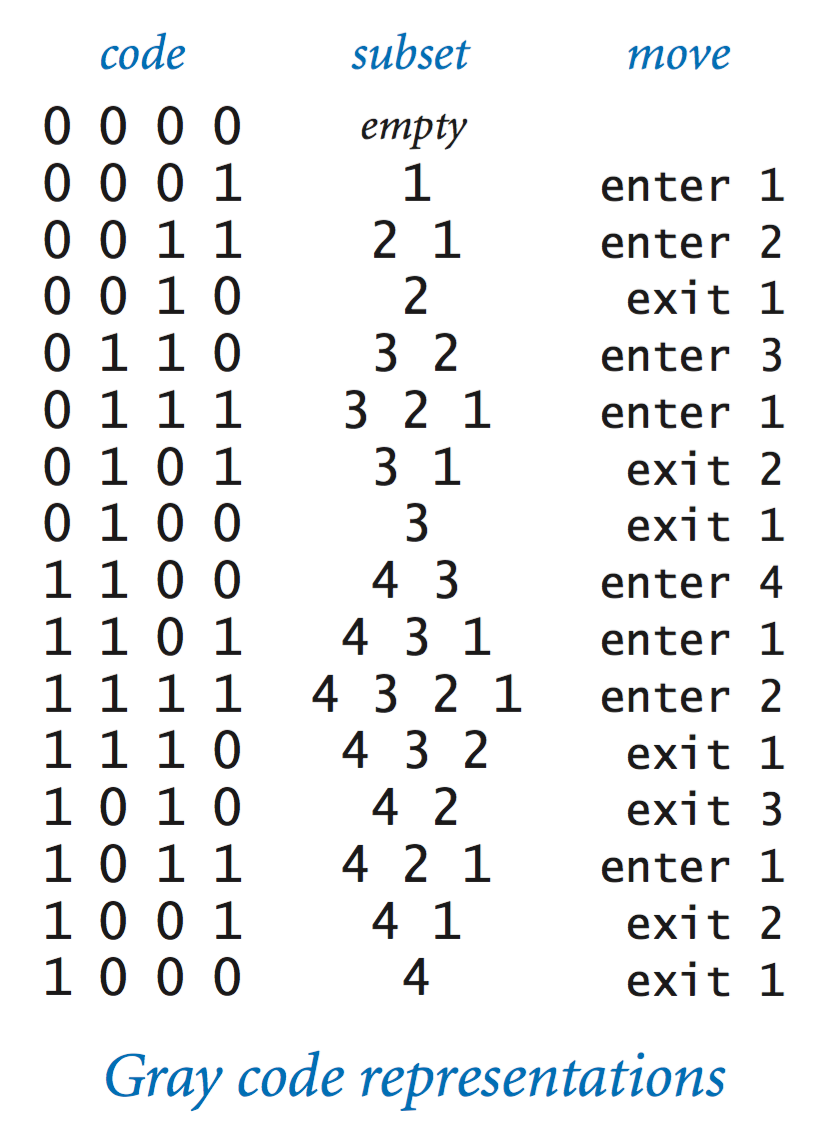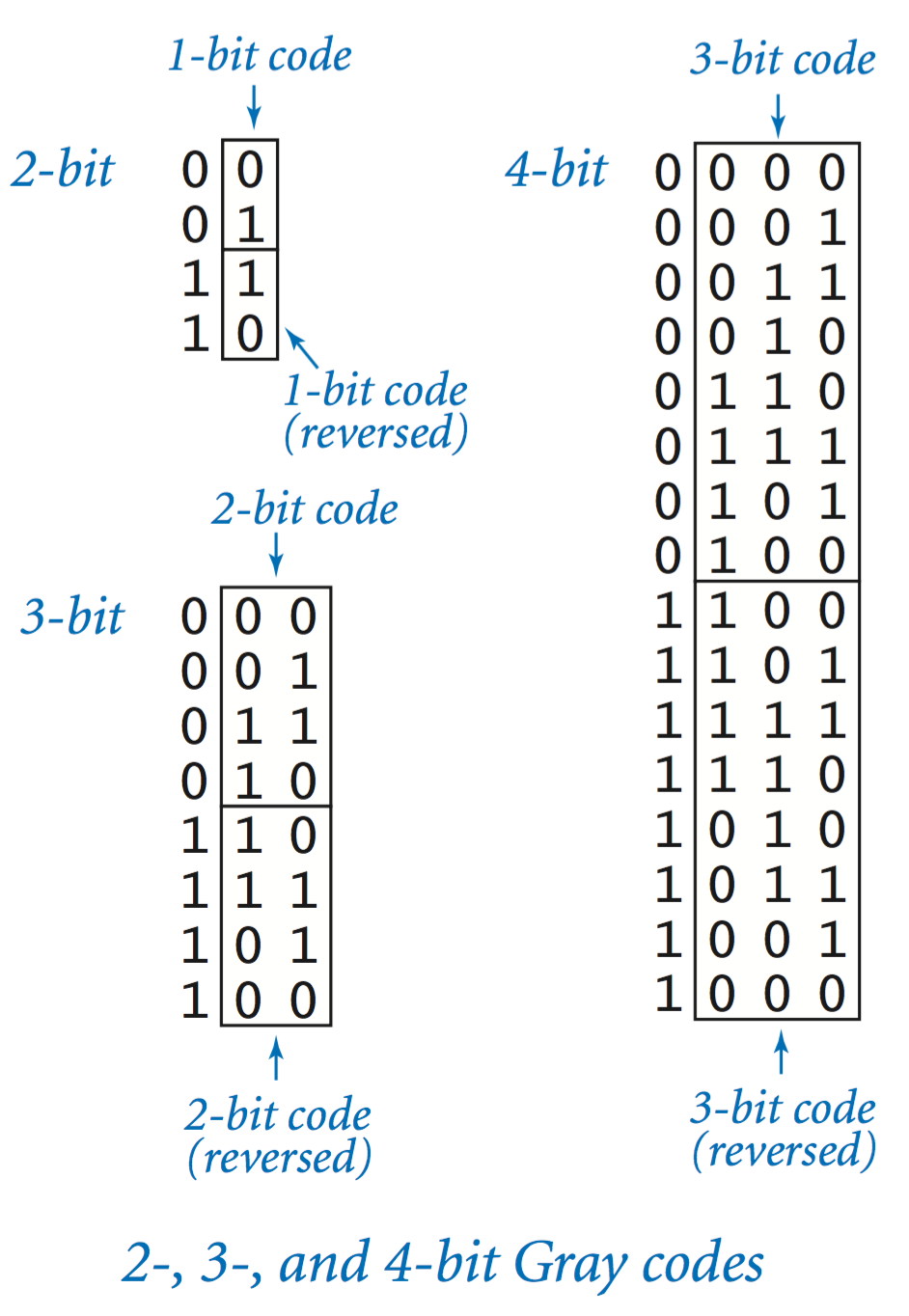Beckett.java uses an n-bit Gray code to print stage directions for an n-character play in such a way that characters enter and exit one at a time so that each subset of characters on the stage appears exactly once.

## Recursive graphics.

Simple recursive drawing schemes can lead to pictures that are remarkably intricate. For example, an H-tree of order n is defined as follows: The base case is null for n = 0. The reduction step is to draw, within the unit square three lines in the shape of the letter H four H-trees of order n − 1, one connected to each tip of the H with the additional provisos that the H-trees of order n − 1 are centered in the four quadrants of the square, halved in size.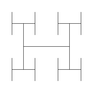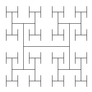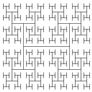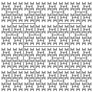Htree.java takes a command-line argument n, and plots to standard drawing an H-tree of order n. An H-tree is a simple example of a fractal: a geometric shape that can be divided into parts, each of which is (approximately) a reduced size copy of the original.

## Brownian bridge.

Brownian.java produces a function graph that approximates a simple example of fractional Brownian motion known as Brownian bridge. You can think of this graph as a random walk that connects the two points (x0, y0) and (x1, y1), controlled by a few parameters. The implementation is based on the midpoint displacement method, which is a recursive plan for drawing the plot within the x-interval [x0, x1]. The base case (when the size of the interval is smaller than a given tolerance) is to draw a straight line connecting the two endpoints. The reduction case is to divide the interval into two halves, proceeding as follows:
• Compute the midpoint (xm, ym) of the interval.
• Add to the y-coordinate ym of the midpoint a random value δ, drawn from the Gaussian distribution with mean 0 and a given variance.
• Recur on the subintervals, dividing the variance by a given scaling factor s.

The shape of the curve is controlled by two parameters: the volatility (initial value of the variance) controls the distance the graph strays from the straight line connecting the points, and the Hurst exponent controls the smoothness of the curve.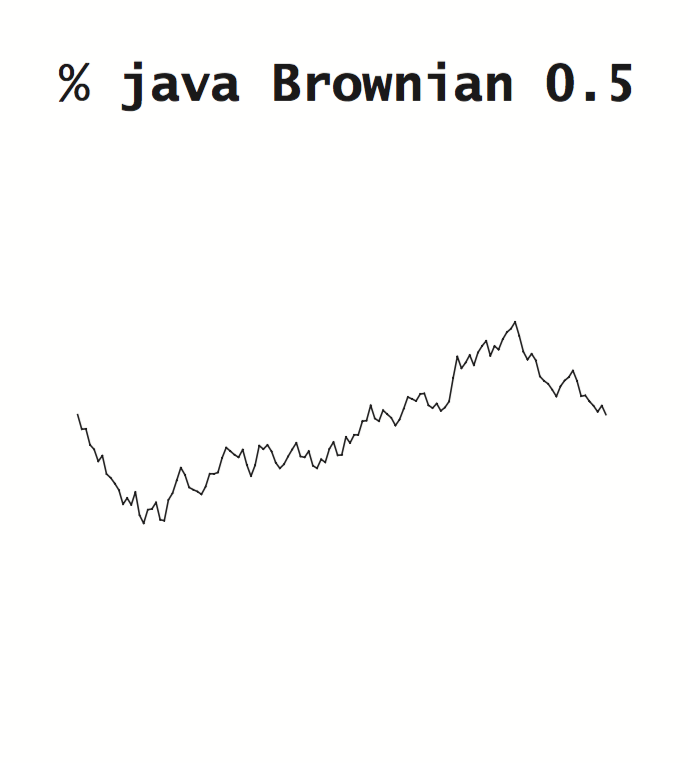## Pitfalls of recursion.

With recursion, you can write compact and elegant programs that fail spectacularly at runtime.
• Missing base case. The recursive function in NoBaseCase.java is supposed to compute harmonic numbers, but is missing a base case:
 public static double harmonic(int n) { return harmonic(n-1) + 1.0/n; } 
If you call this function, it will repeatedly call itself and never return.
• No guarantee of convergence. Another common problem is to include within a recursive function a recursive call to solve a subproblem that is not smaller than the original problem. For example, the recursive function in NoConvergence.java goes into an infinite recursive loop for any value of its argument (except 1).
 public static double harmonic(int n) { if (n == 1) return 1.0; return harmonic(n) + 1.0/n; } 

• Excessive memory requirements. If a function calls itself recursively an excessive number of times before returning, the memory required by Java to keep track of the recursive calls may be prohibitive. The recursive function in ExcessiveMemory.java correctly computes the nth harmonic number. However, calling it with a huge value of n will lead to a StackOverflowError.
 public static double harmonic(int n) { if (n == 0) return 0.0; return harmonic(n-1) + 1.0/n; } 

• Excessive recomputation.The temptation to write a simple recursive program to solve a problem must always be tempered by the understanding that a simple program might require exponential time (unnecessarily), due to excessive recomputation. For example, the Fibonacci sequence
0, 1, 1, 2, 3, 5, 8, 13, 21, 34, 55, 89, 144, 233, ...
is defined by the formula
$$F_n = F_{n-1} + F_{n-2} \text{ for } n \ge 2, \text{ with } F_0 = 0 \text{ and } F_1 = 1$$
A novice programmer might implement this recursive function to compute numbers in the Fibonacci sequence, as in Fibonacci.java:
 // Warning: spectacularly inefficient. public static long fibonacci(int n) { if (n == 0) return 0; if (n == 1) return 1; return fibonacci(n-1) + fibonacci(n-2); } 
However, this program is spectacularly inefficient! To see why it is futile to do so, consider what the function does to compute fibonacci(8) = 21. It first computes fibonacci(7) = 13 and fibonacci(6) = 8. To compute fibonacci(7), it recursively computes fibonacci(6) = 8 again and fibonacci(5) = 5. Things rapidly get worse. The number of times this program computes fibonacci(1) when computing fibonacci(n) is precisely Fn.

## Dynamic programming.

A general approach to implementing recursive programs, The basic idea of dynamic programming is to recursively divide a complex problem into a number of simpler subproblems; store the answer to each of these subproblems; and, ultimately, use the stored answers to solve the original problem. By solving each subproblem only once (instead of over and over), this technique avoids a potential exponential blow-up in the running time.

• Top-down dynamic programming. In top-down dynamic programming, we store or cache the result of each subproblem that we solve, so that the next time we need to solve the same subproblem, we can use the cached values instead of solving the subproblem from scratch. TopDownFibonacci.java illustrates top-down dynamic programming for computing Fibonacci numbers.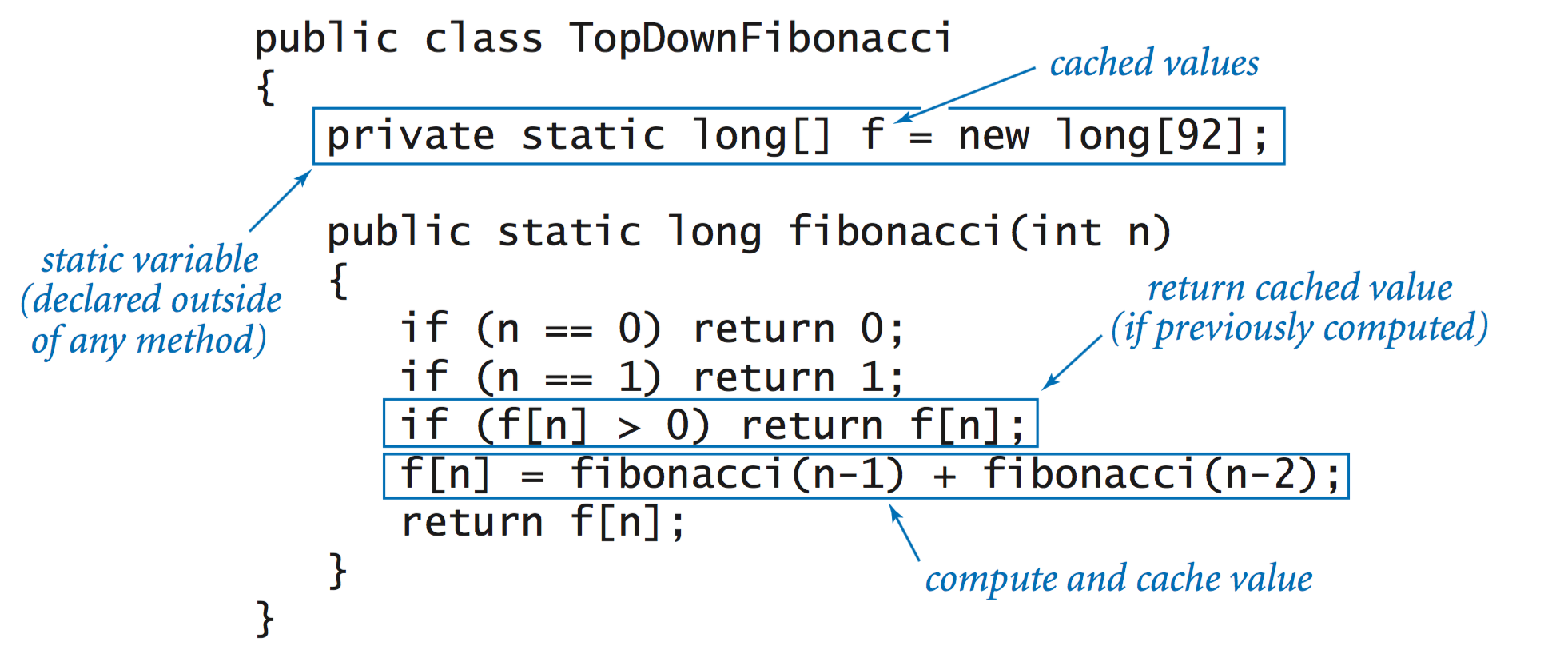• Bottom-up dynamic programming. In bottom-up dynamic programming, we compute solutions to all of the subproblems, starting with the “simplest” subproblems and gradually building up solutions to more and more complicated subproblems. BottomUpFibonacci.java illustrates bottom-up dynamic programming for computing Fibonacci numbers.
 public static long fibonacci(int n) { long[] f = new long[n+1]; f = 0; f = 1; for (int i = 2; i <= n; i++) f[i] = f[i-1] + f[i-2]; return f[n]; } 

• Longest common subsequence problem. Given two strings x and y, we wish to compute their (LCS). If we delete some characters from x and some characters from y, and the resulting two strings are equal, we call the resulting string a common subsequence. The LCS problem is to find a common subsequence of two strings that is as long as possible. For example, the LCS of GGCACCACG and ACGGCGGATACG is GGCAACG, a string of length 7.
 - - G G C - - A - C C A C G A C G G C G G A T - - A C G 

• Longest common subsequence recurrence. Now we describe a recursive formulation that enables us to find the LCS of two given strings s and t. Let m and n be the lengths of s and t, respectively. We use the notation s[i..m) to denote the suffix of s starting at index i, and t[j..n) to denote the suffix of t starting at index j.
• If s and t begin with the same character, then the LCS of s and t contains that first character. Thus, our problem to reduces to finding the LCS of the suffixes s[1..m) and t[1..n).
• If s and t begin with different characters, both characters cannot be part of a common subsequence, so can safely discard one or the other. In either case, the problem reduces to finding the LCS of two strings—either s[0..m) and t[1..n) or s[1..m) and t[0..n).

In general, if we let opt[i][j] denote the length of the LCS of the suffixes s[i..m) and t[j..n), then the following recurrence holds:

 opt[i][j] = 0 if i = m or j = n = opt[i+1][j+1] + 1 if s[i] = t[j] = max(opt[i][j+1], opt[i+1][j]) otherwise 

• Dynamic programming solution. LongestCommonSubsequence.java begins with a bottom-up dynamic programming approach to solving this recurrence.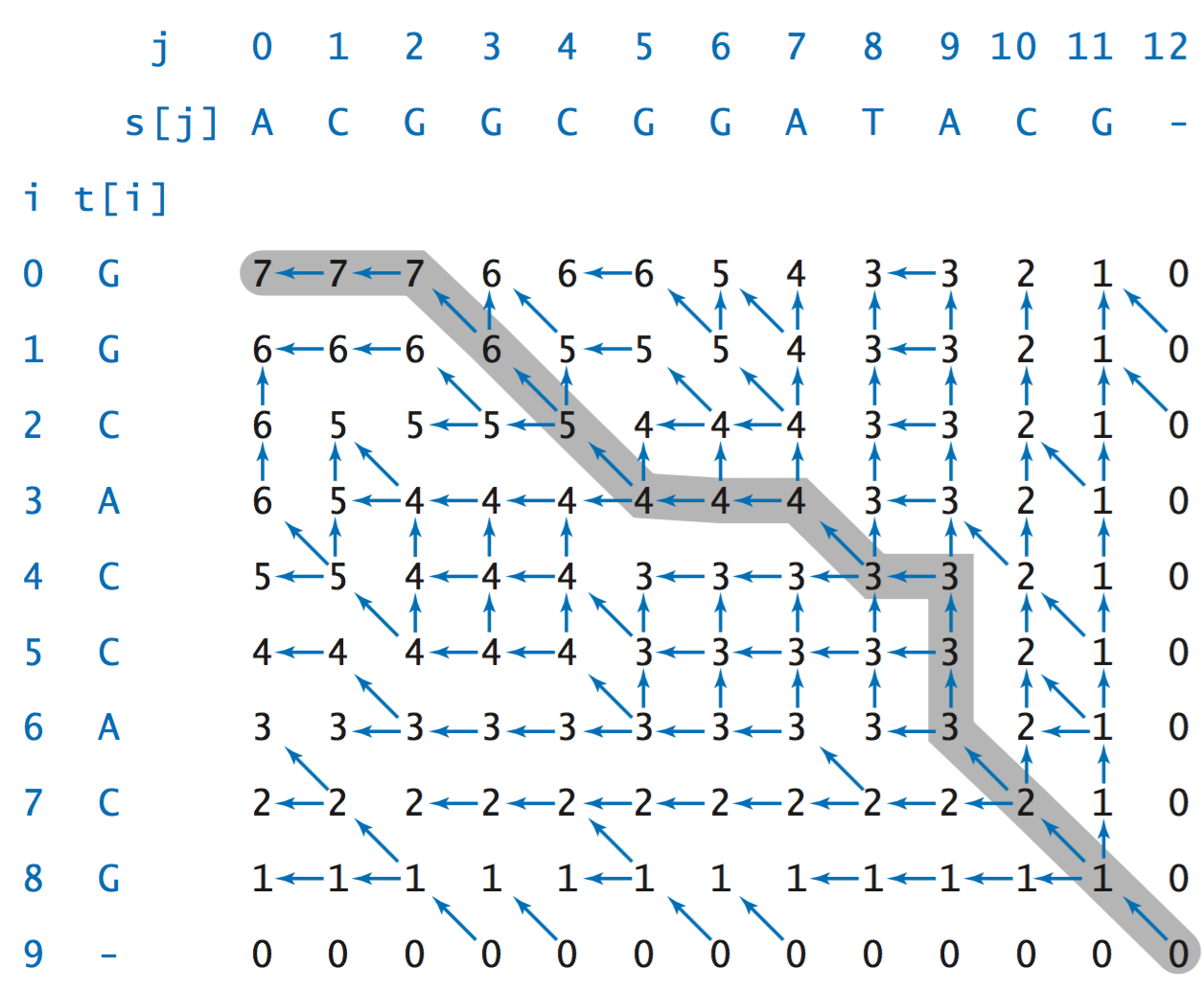The final challenge is to recover the longest common subsequence itself, not just its length. The key idea is to retrace the steps of the dynamic programming algorithm backward, rediscovering the path of choices (highlighted in gray in the diagram) from opt to opt[m][n]. To determine the choice that led to opt[i][j], we consider the three possibilities:

• The character s[i] matches t[j]. In this case, we must have opt[i][j] = opt[i+1][j+1] + 1, and the next character in the LCS is s[i]. We continue tracing back from opt[i+1][j+1].

• The LCS does not contain s[i]. In this case, opt[i][j] = opt[i+1][j] and we continue tracing back from opt[i+1][j].

• The LCS does not contain t[j]. In this case, opt[i][j] = opt[i][j+1] and we continue tracing back from opt[i][j+1].

#### Exercises

1. Given four positive integers a, b, c, and d, explain what value is computed by gcd(gcd(a, b), gcd(c, d)).

Solution: the greatest common divisor of a, b, c, and d.

2. Explain in terms of integers and divisors the effect of the following Euclid-like function.
 public static boolean gcdlike(int p, int q) { if (q == 0) return (p == 1); return gcdlike(q, p % q); } 

Solution: Returns whether p and q are relatively prime.

3. Consider the following recursive function.
 public static int mystery(int a, int b) { if (b == 0) return 0; if (b % 2 == 0) return mystery(a+a, b/2); return mystery(a+a, b/2) + a; } 

What are the values of mystery(2, 25) and mystery(3, 11)? Given positive integers a and b, describe what value mystery(a, b) computes. Answer the same question, but replace + with * and replace return 0 with return 1.

Solution: 50 and 33. It computes a*b. If you replace + with *, it computes a^b.

4. Write a program AnimatedHtree.java that animates the drawing of the H-tree.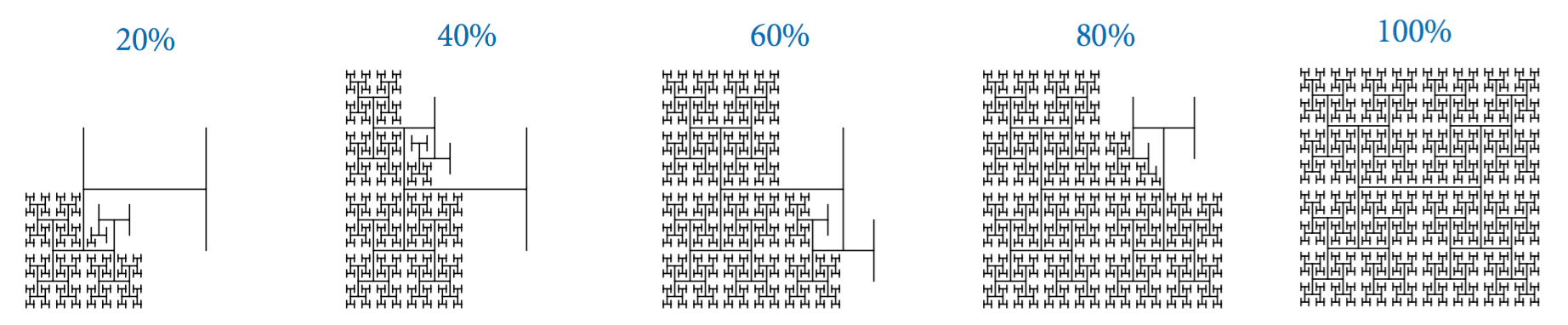Next, rearrange the order of the recursive calls (and the base case), view the resulting animation, and explain each outcome.

#### Creative Exercises

1. Binary representation. Write a program IntegerToBinary.java that takes a positive integer n (in decimal) as a command-line argument and prints its binary representation. Recall, in Binary.java, we used the method of subtracting out powers of 2. Now, use the following simpler method: repeatedly divide 2 into n and read the remainders backwards. First, write a while loop to carry out this computation and print the bits in the wrong order. Then, use recursion to print the bits in the correct order.
2. Permutations. Write a program Permutations.java that take an integer command-line argument n and prints all n! permutations of the n letters starting at a (assume that n is no greater than 26). A permutation of n elements is one of the n! possible orderings of the elements. As an example, when n = 3 you should get the following output (but do not worry about the order in which you enumerate them):
 bca cba cab acb bac abc 
3. Permutations of size k. Write a program PermutationsK.java that two command-line arguments n and k, and prints out all $$P(n, k) = \frac{n!}{(n-k)!}$$ permutations that contain exactly k of the n elements. Below is the desired output when k = 2 and n = 4 (again, do not worry about the order):
 ab ac ad ba bc bd ca cb cd da db dc 
4. Combinations. Write a program Combinations.java that takes an integer command-line argument n and prints all 2n combinations of any size. A combination is a subset of the n elements, independent of order. As an example, when n = 3, you should get the following output:
  a ab abc ac b bc c 
Note that your program needs to print the empty string (subset of size 0).
5. Combinations of size k. Write a program CombinationsK.java that takes two command-line arguments n and k, and prints all $$C(n, k) = \frac{n!}{k! (n-k)!}$$ combinations of size k. For example, when n = 5 and k = 3, you should get the following output:
 abc abd abe acd ace ade bcd bce bde cde 
Alternate solution using arrays instead of strings: Comb2.java.
6. Recursive squares. Write a program to produce each of the following recursive patterns. The ratio of the sizes of the squares is 2.2:1. To draw a shaded square, draw a filled gray square, then an unfilled black square.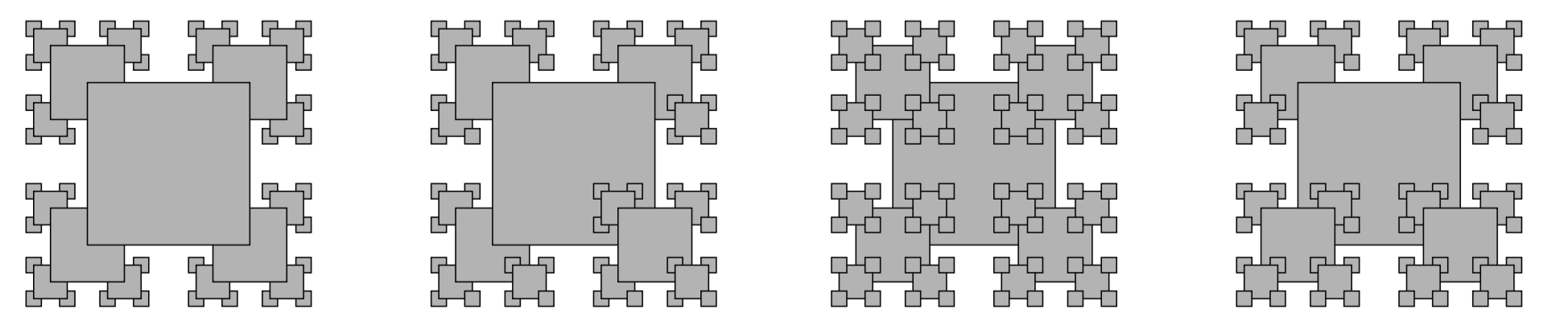RecursiveSquares.java gives a solution to the first pattern.

7. Gray code. Modify Beckett.java to print the Gray code (not just the sequence of bit positions that change).

Solution: GrayCode.java uses Java's string data type; GrayCodeArray.java uses a boolean array.

8. Animated towers of Hanoi animation. Write a program AnimatedHanoi.java that uses StdDraw to animate a solution to the towers of Hanoi problem, moving the discs at a rate of approximately 1 per second.
9. Collatz function. Consider the following recursive function in Collatz.java, which is related to a famous unsolved problem in number theory, known as the Collatz problem or the 3n + 1 problem.
 public static void collatz(int n) { StdOut.print(n + " "); if (n == 1) return; if (n % 2 == 0) collatz(n / 2); else collatz(3*n + 1); } 

For example, a call to collatz(7) prints the sequence

7 22 11 34 17 52 26 13 40 20 10 5 16 8 4 2 1

as a consequence of 17 recursive calls. Write a program that takes a command-line argument n and returns the value of i < n for which the number of recursive calls for collatz(i) is maximized. Hint: use memoization. The unsolved problem is that no one knows whether the function terminates for all integers (mathematical induction is no help because one of the recursive calls is for a larger value of the argument).
10. Brownian island. B. Mandelbrot asked the famous question How long is the coast of Britain? Modify Brownian.java to get a program BrownianIsland.java that plots Brownian islands, whose coastlines resemble that of Great Britain. The modifications are simple: first, change curve() to add a random Gaussian to the x-coordinate as well as to the y-coordinate; second, change main() to draw a curve from the point at the center of the canvas back to itself. Experiment with various values of the arguments to get your program to produce islands with a realistic look.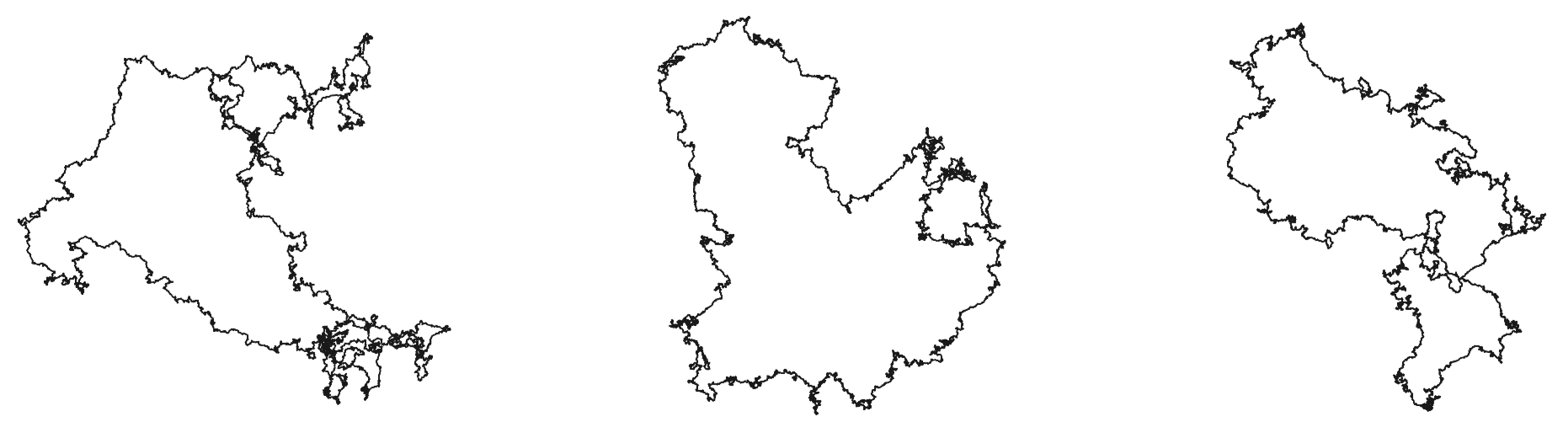11. Plasma clouds. Write a recursive program PlasmaCloud.java to draw plasma clouds, using the method suggested in the text.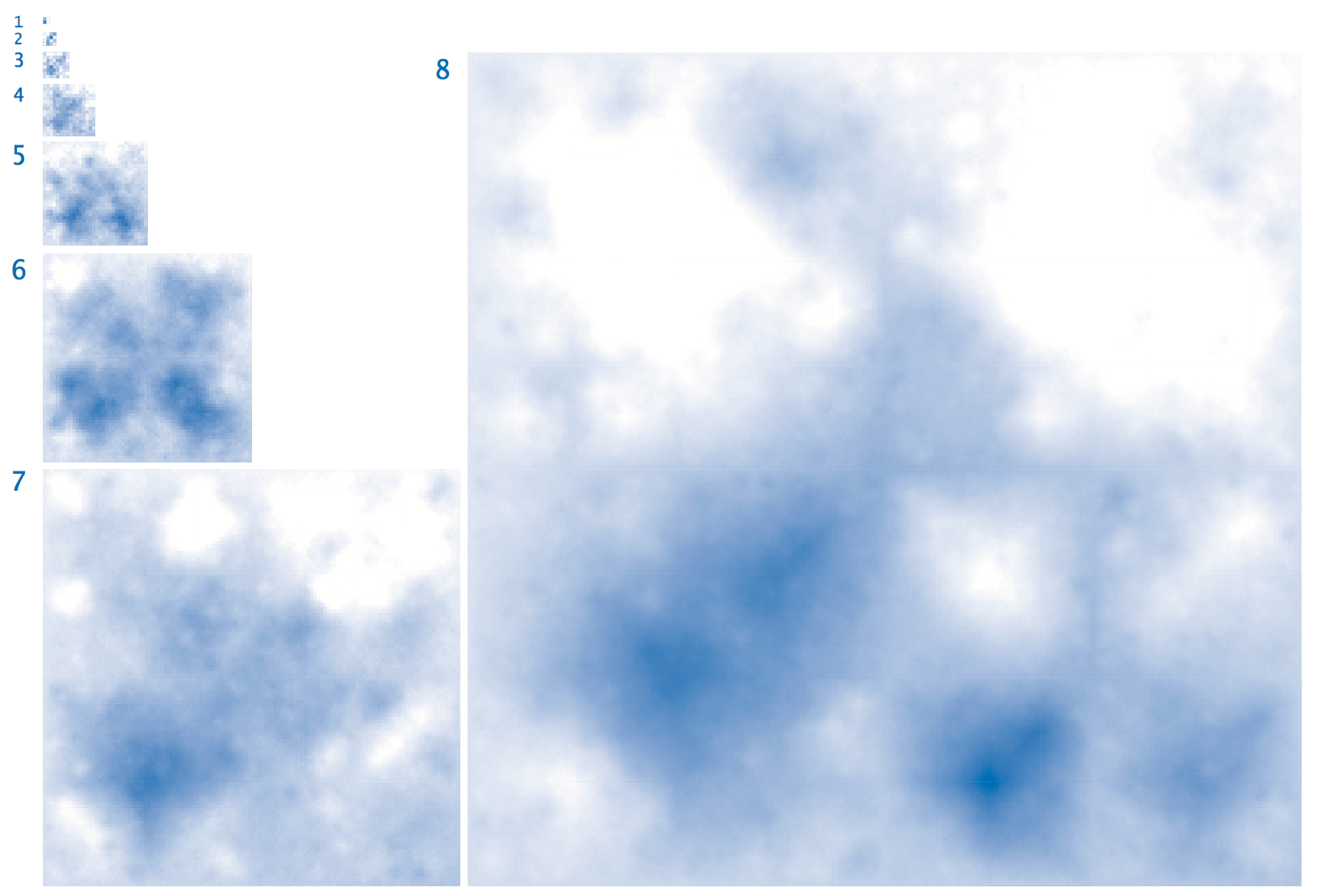12. A strange function. Consider McCarthy's 91 function:
 public static int mcCarthy(int n) { if (n > 100) return n - 10; else return mcCarthy(mcCarthy(n+11)); } 

Determine the value of mcCarthy(50) without using a computer. Give the number of recursive calls used by mcCarthy() to compute this result. Prove that the base case is reached for all positive integers n or find a value of n for which this function goes into a recursive loop.

13. Recursive tree. Write a program Tree.java that takes a command-line argument n and produces the following recursive patterns for n equal to 1, 2, 3, 4, and 8.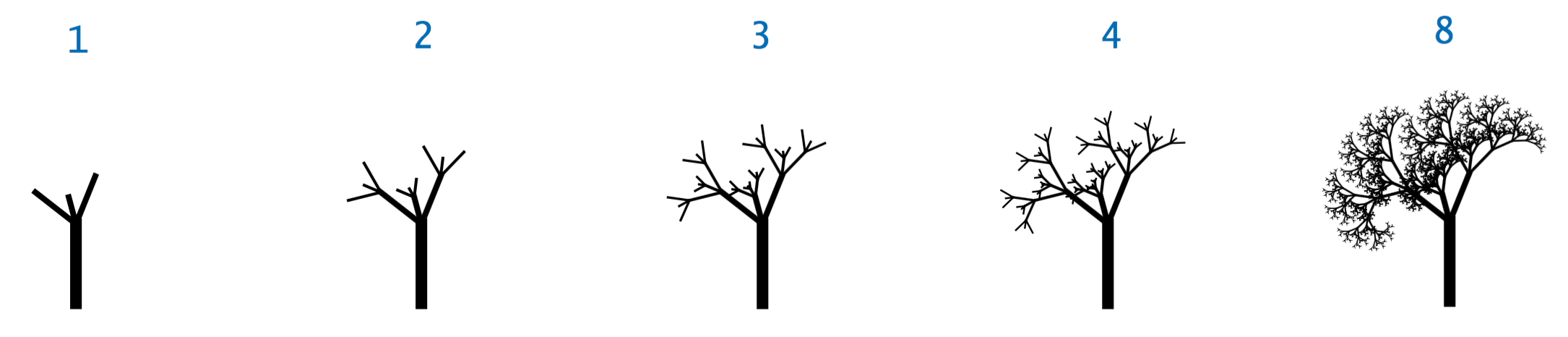#### Web Exercises

1. Does Euclid.java still work if the inputs can be negative? If not, fix it. Hint: Recall that % can return a negative integer if the first input is negative. When calling the function, take the absolute value of both inputs.
2. Write a recursive program GoldenRatio.java that takes an integer input N and computes an approximation to the golden ratio using the following recursive formula:
 f(N) = 1 if N = 0 = 1 + 1 / f(N-1) if N > 0 
Redo, but do not use recursion.
3. Discover a connection between the golden ratio and Fibonacci numbers. Hint: consider the ratio of successive Fibonacci numbers: 2/1, 3/2, 8/5, 13/8, 21/13, 34/21, 55/34, 89/55, 144/89, ...
4. Consider the following recursive function. What is mystery(1, 7)?
 public static int mystery(int a, int b) { if (0 == b) return 0; else return a + mystery(a, b-1); } 

Will the function in the previous exercise terminate for every pair of integers a and b between between 0 and 100? Give a high level description of what mystery(a, b) returns, given integers a and b between 0 and 100.

Answer: mystery(1, 7) = 1 + mystery(1, 6) = 1 + (1 + mystery(1, 5)) = ... 7 + mystery(1, 0) = 7.

Answer: Yes, the base case is b = 0. Successive recursive calls reduce b by 1, driving it toward the base case. The function mystery(a, b) returns a * b. Mathematically inclined students can prove this fact via induction using the identity ab = a + a(b-1).

5. Consider the following function. What does mystery(0, 8) do?
 public static void mystery(int a, int b) { if (a != b) { int m = (a + b) / 2; mystery(a, m); StdOut.println(m); mystery(m, b); } } 
6. Consider the following function. What does mystery(0, 8) do?
 public static void mystery(int a, int b) { if (a != b) { int m = (a + b) / 2; mystery(a, m - 1); StdOut.println(m); mystery(m + 1, b); } } 
7. Repeat the previous exercise, but replace if (a != b) with if (a <= b).
8. What does mystery(0, 8) do?
 public static int mystery(int a, int b) { if (a == b) StdOut.println(a); else { int m1 = (a + b ) / 2; int m2 = (a + b + 1) / 2; mystery(a, m1); mystery(m2, b); } } 
9. What does the following function compute?
 public static int f(int n) { if (n == 0) return 0; if (n == 1) return 1; if (n == 2) return 1; return 2*f(n-2) + f(n-3); 
10. Write a program Fibonacci2.java that takes a command-line argument N and prints out the first N Fibonacci numbers using the following alternate definition:
 F(n) = 1 if n = 1 or n = 2 = F((n+1)/2)2 + F((n-1)/2)2 if n is odd = F(n/2 + 1)2 - F(n/2 - 1)2 if n is even 

What is the biggest Fibonacci number you can compute in under a minute using this definition? Compare this to Fibonacci.java.

11. Write a program that takes a command-line argument N and prints out the first N Fibonacci numbers using the following method proposed by Dijkstra:
 F(0) = 0 F(1) = 1 F(2n-1) = F(n-1)^2 + F(n)^2 F(2n) = (2F(n-1) + F(n)) * F(n) 
12. Prove by mathematical induction that the alternate definitions of the Fibonacci function given in the previous two exercises are equivalent to the original definition.
13. Write a program Pell.java that takes a command-line argument N and prints out the first N Pell numbers: p0 = 0, p1 = 1, and for n >= 2, pn = 2 pn-1 + pn-2. Print out the ratio of successive terms and compare to 1 + sqrt(2).
14. Consider the following function from program Recursion.java:
 public static void mystery(int n) { if (n == 0 || n == 1) return; mystery(n-2); StdOut.println(n); mystery(n-1); } 

What does mystery(6) print out? Hint: first figure out what mystery(2), mystery(3), and so forth print out.

15. What would happen in the previous exercise if the base case was replaced with the following statement?
 if (n == 0) return; 
16. Consider the following recursive functions.
 public static int square(int n) { if (n == 0) return 0; return square(n-1) + 2*n - 1; } public static int cube(int n) { if (n == 0) return 0; return cube(n-1) + 3*(square(n)) - 3*n + 1; } 

What is the value of square(5)? cube(5)? cube(123)?

17. Consider the following pair of mutually recursive functions. What does g(g(2)) evaluate to?
 public static int f(int n) { public static int g(int n) { if (n == 0) return 0; if (n == 0) return 0; return f(n-1) + g(n-1); return g(n-1) + f(n); } } 
18. Write program to verify that (for small values of n) the sum of the cubes of the first n Fibonacci numbers F(0)^3 + F(1)^3 + ... + F(n)^3 equals (F(3n+4) + (-1)^n * 6 * f(n-1)) / 10, where F(0) = 1, F(1) = 1, F(2) = 2, and so forth.
19. Transformations by increment and unfolding. Given two integers a ≤ b, write a program Sequence.java that transforms a into b by a minimum sequence of increment (add 1) and unfolding (multiply by 2) operations. For example,
 % java Sequence 5 23 23 = ((5 * 2 + 1) * 2 + 1) % java Sequence 11 113 113 = ((((11 + 1) + 1) + 1) * 2 * 2 * 2 + 1) 
20. Hadamard matrix. Write a recursive program Hadamard.java that takes a command-line argument n and plots an N-by-N Hadamard pattern where N = 2n. Do not use an array. A 1-by-1 Hadamard pattern is a single black square. In general a 2N-by-2N Hadamard pattern is obtained by aligning 4 copies of the N-by-N pattern in the form of a 2-by-2 grid, and then inverting the colors of all the squares in the lower right N-by-N copy. The N-by-N Hadamard H(N) matrix is a boolean matrix with the remarkable property that any two rows differ in exactly N/2 bits. This property makes it useful for designing error-correcting codes. Here are the first few Hadamard matrices.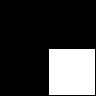21. 8 queens problem. In this exercise, you will solve the classic 8-queens problem: place 8 queens on an 8-by-8 chess board so that no two queens are in the same row, column, or diagonal. There are 8! = 40,320 ways in which no two queens are placed in the same row or column. Any permutation p[] of the integers 0 to 7 gives such a placement: put queen i in row i, column p[i]. Your program Queens.java should take an integer command-line argument n and enumerate all solutions to the n-queens problem by drawing the location of the queens in ASCII like the two solutions below.
 * * * Q * * * * * * * * Q * * * * Q * * * * * * * Q * * * * * * * * * * * * Q * * * * Q * * * * * * Q * * * * * * * * * * * Q * * * * * * Q * * * * Q * * * * * * * * * * * * Q * * * * * * * Q * * * * Q * * * * * * * * Q * * Q * * * * * * * Q * * * * * * * 
Hint: to determine whether setting q[n] = i conflicts with q through q[n-1]
• if q[i] equals q[n]: two queens are placed in the same column
• if q[i] - q[n] equals n - i: two queens are on same major diagonal
• if q[n] - q[i] equals n - i: two queens are on same minor diagonal
22. Another 8 queens solver. Program Queens2.java solves the 8 queens problem by implicitly enumeration all n! permutations (instead of the n^n placements). It is based on program Permutations.java.
23. Euclid's algorithm and π. The probability that two numbers chosen from a large random set of numbers have no common factors (other than 1) is 6 / π2. Use this idea to estimate π. Robert Matthews use the same idea to estimate π by taken the set of numbers to be a function of the positions of stars in the sky.
24. Towers of Hanoi variant II. (Knuth-Graham and Pathashnik) Solve the original Towers of Hanoi problem, but with the extra restriction that you are not allowed to directly transfer a disk from A to C. How many moves does it take to solve a problem with n disks? Hint: move n-1 smallest disks from A to C recursively (without any direct A to C moves), move disk n from A to B, move n-1 smallest disks from C to A (without any direct A to C moves), move disk N from B to C, and move n-1 smallest disks from A to C recursively (without any direct A to C moves).
25. Towers of Hanoi variant III. Repeat the previous question but disallow both A to C and C to A moves. That is, each move must involve pole B.
26. Towers of Hanoi with 4 pegs. Suppose that you have a fourth peg. What is the least number of moves needed to transfer a stack of 8 disks from the leftmost peg to the rightmost peg? Answer. Finding the shortest such solution in general has remained an open problem for over a hundred years and is known as Reve's puzzle.
27. Another tricky recursive function. Consider the following recursive function. What is f(0)?
 public static int f(int x) { if (x > 1000) return x - 4; else return f(f(x+5)); } 
28. Checking if n is a Fibonacci number. Write a function to check if n is a Fibonacci number. Hint: a positive integer is a Fibonacci number if and only if either (5*n*n + 4) or (5*n*n - 4) is a perfect square.
29. Random infix expression generator. Run RandomExpression.java with different command-line argument p between 0 and 1. What do you observe?
 public static String expr(double p) { double r = Math.random(); if (r <= 1*p) return "(" + expr(p) + " + " + expr(p) + ")"; if (r <= 2*p) return "(" + expr(p) + " * " + expr(p) + ")"; return "" + (int) (100 * Math.random()); } 
30. A tricky recurrence. Define F(n) so that F(0) = 0 and F(n) = n - F(F(n-1)). What is F(100000000)?

Solution: The answer is related to the Fibonacci sequence and the Zeckendorf representation of a number.

31. von Neumann ordinal. The von Neumann integer i is defined as follows: for i = 0, it is the empty set; for i > 0, it is the set containing the von Neumann integers 0 to i-1.
 0 = {} = {} 1 = {0} = {{}} 2 = {0, 1} = {{}, {{}}} 3 = {0, 1, 2} = {{}, {{}}, {{}, {{}}}} 
Write a program Ordinal.java with a recursive function vonNeumann() that takes a nonnegative integer N and returns a string representation of the von Neumann integer N. This is a method for defining ordinals in set theory.
32. Subsequences of a string. Write a program Subsequence.java that takes a string command-line argument s and an integer command-line argument k and prints out all subsequences of s of length k.
 % java Subsequence abcd 3 abc abd acd bcd 
33. Interleaving two strings. Given two strings s and t of distinct characters, print out all (M+N)! / (M! N!) interleavings, where M and N are the number of characters in the two strings. For example, if
 s = "ab" t = "CD" abCD CabD aCbD CaDb aCDb CDab 
34. Binary GCD. Write a program BinaryGCD.java that finds the greatest common divisor of two positive integers using the binary gcd algorithm: gcd(p, q) =
• p if q = 0
• q if p = 0
• 2 * gcd(p/2, q/2) if p and q are even
• gcd(p/2, q) if p is even and q is odd
• gcd(p, q/2) if p is odd and q is even
• gcd((p-q)/2, q) if p and q are odd and p >= q
• gcd(p, (q-p)/2) if p and q are odd and p < q
35. Integer partitions. Write a program Partition.java that takes a positive integer N as a command-line argument and prints out all partitions of N. A partition of N is a way to write N as a sum of positive integers. Two sums are considered the same if they only differ in the order of their constituent summands. Partitions arise in symmetric polynomials and group representation theory in mathematics and physics.
 % java Partition 4 % java Partition 6 4 6 3 1 5 1 2 2 4 2 2 1 1 4 1 1 1 1 1 1 3 3 3 2 1 3 1 1 1 2 2 2 2 2 1 1 2 1 1 1 1 1 1 1 1 1 1 
36. Johnson-Trotter permutations. Write a program JohnsonTrotter.java that takes an integer command-line argument n and prints all n! permutations of the integer 0 through n-1 in such a way that consecutive permutations differ in only one adjacent transposition (similar to way Gray code iterates over combinations in such a way that consecutive combinations differ in only one bit).
 % java JohnsonTrotter 3 012 (2 1) 021 (1 0) 201 (2 1) 210 (0 1) 120 (1 2) 102 (0 1) 
37. Permutations in lexicographic order. Write a program PermutationsLex.java that take a command-line argument N and prints out all N! permutations of the integer 0 through N-1 in lexicographic order.
 % java PermutationsLex 3 012 021 102 120 201 210 
38. Derangements. A derangement is a permutation p[] of the integers from 0 to N-1 such that p[i] doesn't equal i for any i. For example there are 9 derangements when N = 4: 1032, 1230, 1302, 2031, 2301, 2310, 3012, 3201, 3210. Write a program to count the number of derangements of size N using the following recurrence: d[N] = (N-1) (d[N-1] + d[N-2]), where d = 0, d = 1. The first few terms are 0, 1, 2, 9, 44, 265, 1854, 14833, 133496, and 1334961.
39. Tribonacci numbers. The tribonacci numbers are similar to the Fibonacci numbers, except that each term is the sum of the three previous terms in the sequence. The first few terms are 0, 0, 1, 1, 2, 4, 7, 13, 24, 44, 81. Write a program to compute tribonacci numbers. What is the ratio successive terms? Answer. Root of x^3 - x^2 - x - 1, which is approximately 1.83929.
40. Sum of first n Fibonacci numbers. Prove by induction that the sum of the first n Fibonacci numbers F(1) + F(2) + ... + F(N) is F(N+2) - 1.
41. Combinational Gray code. Print out all combination of k of n items in such a way that consecutive combinations differ in exactly one element, e.g., if k = 3 and n = 5, 123, 134, 234, 124, 145, 245, 345, 135, 235, 125. Hint: use the Gray code, but only print out those integers with exactly k 1's in their binary representation.

42. Maze generation. Create a maze using divide-and-conquer: Begin with a rectangular region with no walls. Choose a random gridpoint in the rectangle and construct two perpendicular walls, dividing the square into 4 subregions. Choose 3 of the four regions at random and open a one cell hole at a random point in each of the 3. Recur until each subregion has width or height 1.

43. Plasma clouds. Program PlasmaCloud.java takes a command-line argument N and produces a random N-by-N plasma fractal using the midpoint displacement method.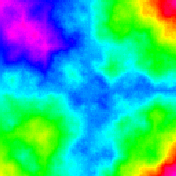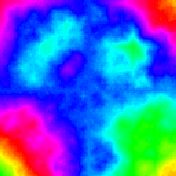Here's an 800-by-800 example. Here's a reference, including a simple 1D version. Note: some visual artifacts are noticeable parallel to the x and y axes. Doesn't have all of the statistical properties of 2D fractional Brownian motion.

44. Fern fractal. Write a recursive program to draw a fern or tree, as in this fern fractal demo.
45. Integer set partition. Use memoization to develop a program that solves the set partition problem for positive integer values. You may use an array whose size is the sum of the input values.
46. Voting power. John F. Banzhaf III proposed a ranking system for each coalition in a block voting system. Suppose party i control w[i] votes. A strict majority of the votes is needed to accept or reject a proposal. The voting power of party i is the number of minority coalitions it can join and turn it into a winning majority coalition. Write a program VotingPower.java that takes in a list of coalition weights as command-line argument and prints out the voting power of each coalition. Hint: use Schedule.java as a starting point.
47. Scheduling on two parallel machines. Program Schedule.java takes a command-line argument N, reads in N real number of standard input, and partitions them into two groups so that their difference is minimized.
48. Hofstadter–Conway $10,000 sequence. Consider the following recursive function. f(n) = f(f(n-1)) + f(n-f(n-1)) for n > 2 and f(1) = f(2) = 1. Compute f(3). Write a Java program to compute the first 50 values of f(n) in the Hofstadter–Conway$10,000 sequence. Use dynamic programming. This sequence has many fascinating properties and connects with Pascal's triangle, the Gaussian distribution, Fibonacci numbers, and Catalan numbers.
49. Running time recurrences. Use dynamic programming to compute a table of values T(N), where T(N) is the solution to the following divide-and-conquer recurrence. T(1) = 0, T(N) = N + T(N/2) + T(N - N/2) if N > 1.
50. Gas station optimization. You are driving from Princeton to San Francisco in a car that gets 25 miles per gallon and has a gas tank capacity of 15 gallons. Along the way, there are N gas stations where you can stop for gas. Gas station i is d[i] miles into the trip and sells gas for p[i] dollars per gallon. If you stop at station i for gas, you must completely fill up your tank. Assume that you start with a full tank and that the d[i] are integers. Use dynamic programming to find a minimum cost sequence of stops.
51. Unix diff. The Unix diff program compares two files line-by-line and prints out places where they differ. Write a program Diff.java that reads in two files specified at the command line one line at a time, computes the LCS on the sequence of constituent lines of each file, and prints out any lines corresponding to non-matches in the LCS.
52. Longest common subsequence of 3 strings. Given 3 strings, find the longest common subsequence using dynamic programming. What is the running time and memory usage of your algorithm?
53. Making change. Given A hundred dollar bills, B fifty dollar bills, C twenty dollar bills, D ten dollar bills, E five dollar bills, F one dollar bills, G half-dollars, H quarters, I dimes, J nickels, and K pennies, determine whether it is possible to make change for N cents. Hint: knapsack problem. (Greedy also works.)
54. Making change. Suppose that you are a cashier in a strange country where the currency denominations are: 1, 3, 8, 16, 22, 57, 103, and 526 cents (or more generally d0, d1, ..., dN-1. Describe a dynamic programming algorithm to make change for c cents using the fewest number of coins. Hint: the greedy algorithm won't work since the best way to change 114 cents is 57 + 57 instead of 103 + 8 + 3.
55. Longest increasing sequence. Given an array of N 64-bit integers, find the longest subsequence that is strictly increasing.

Hint. Compute the longest common subsequence between the original array and a sorted version of the array where duplicate copies of an integer are removed.

56. Longest common increasing sequence. Computational biology. Given two sequences of N 64-bit integers, find the longest increasing subsequence that is common to both sequences.
57. Activity selection with profits. Job i has start time s_i, finish time f_i and profit p_i. Find best subset of jobs to schedule.
58. Diff. Write a program that reads in two files and prints out their diff. Treat each line as a symbol and compute an LCS. Print out those lines in each file that aren't in the LCS.
59. Knapsack problem. Knapsack.java.
60. Text justification. Write a program that takes a command line argument N, reads text from standard input, and prints out the text, formatted nicely with at most N characters per line. Use dynamic programming.
61. Viterbi algorithm. Given a directed graph where each edge is labeled with a symbol from a finite alphabet. Is there a path from one distinguished vertex x that matches the characters in the string s? Dynamic programming. A(i, v) = 0 or 1 if there is a path from x to v that consumes the first i characters of s. A(i, v) = max (A(i-1, u) : (u, v) in E labeled with s[i]).
62. Viterbi algorithm. Speech recognition, handwriting analysis, computational biology, hidden Markov models. Suppose each edge leaving v has a probability p(v, w) of being traversed. Probability of a path is the product of the probability on that path. What is most probable path? Dynamic programming.
63. Smith–Waterman algorithm. Local sequence alignment.

64. Binomial coefficients (brute-force). The binomial coefficient C(n, k) is the number of ways of choosing a subset of k elements from a set of n elements. It arises in probability and statistics. One formula for computing binomial coefficients is C(n, k) = n! / (k! (n-k)!). This formula is not so amenable to direct computation because the intermediate results may overflow, even if the final answer does not. For example C(100, 15) = 253338471349988640 fits in a 64-bit long, but the binary representation of 100! is 525 bits long.

Pascal's identity expresses C(n, k) in terms of smaller binomial coefficients: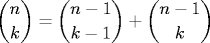SlowBinomial.java fails spectacularly for medium n or k, not because of overflow, but rather because the same subproblems are solved repeatedly.

 // DO NOT RUN THIS CODE FOR LARGE INPUTS public static long binomial(int n, int k) { if (k == 0) return 1; if (n == 0) return 0; return binomial(n-1, k) + binomial(n-1, k-1); } 

65. Binomial coefficients (dynamic programming). Write a program Binomial.java that takes two command-line arguments n and k and uses bottom-up dynamic programming to compute C(n, k).